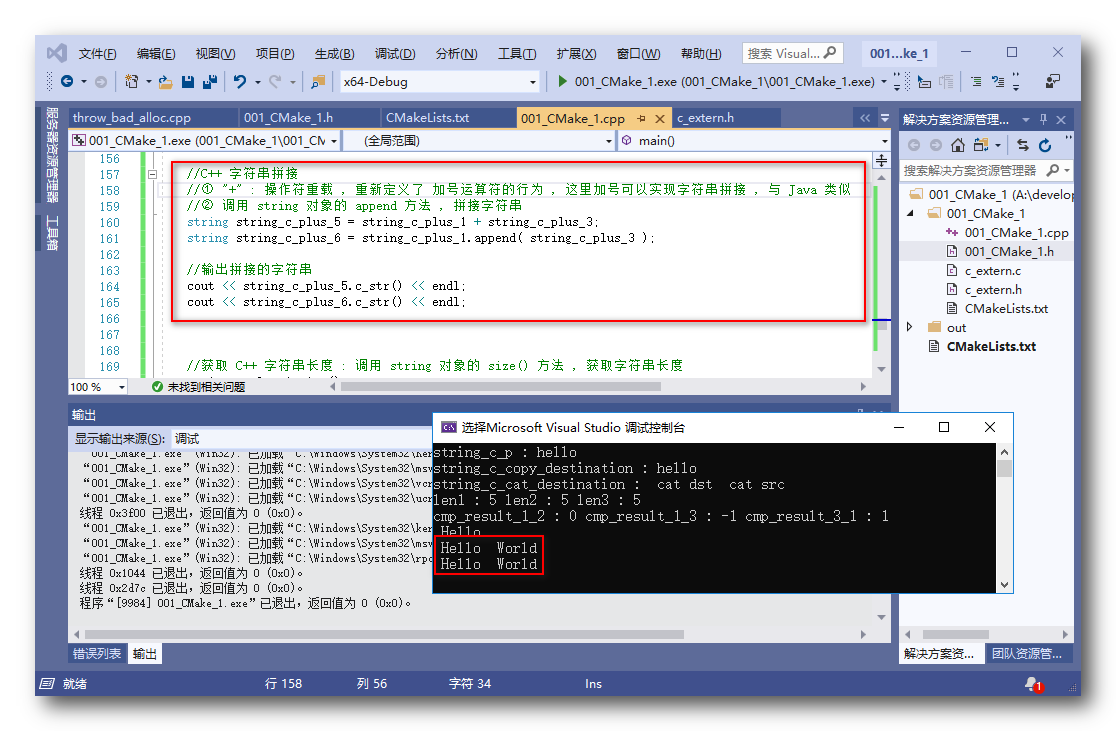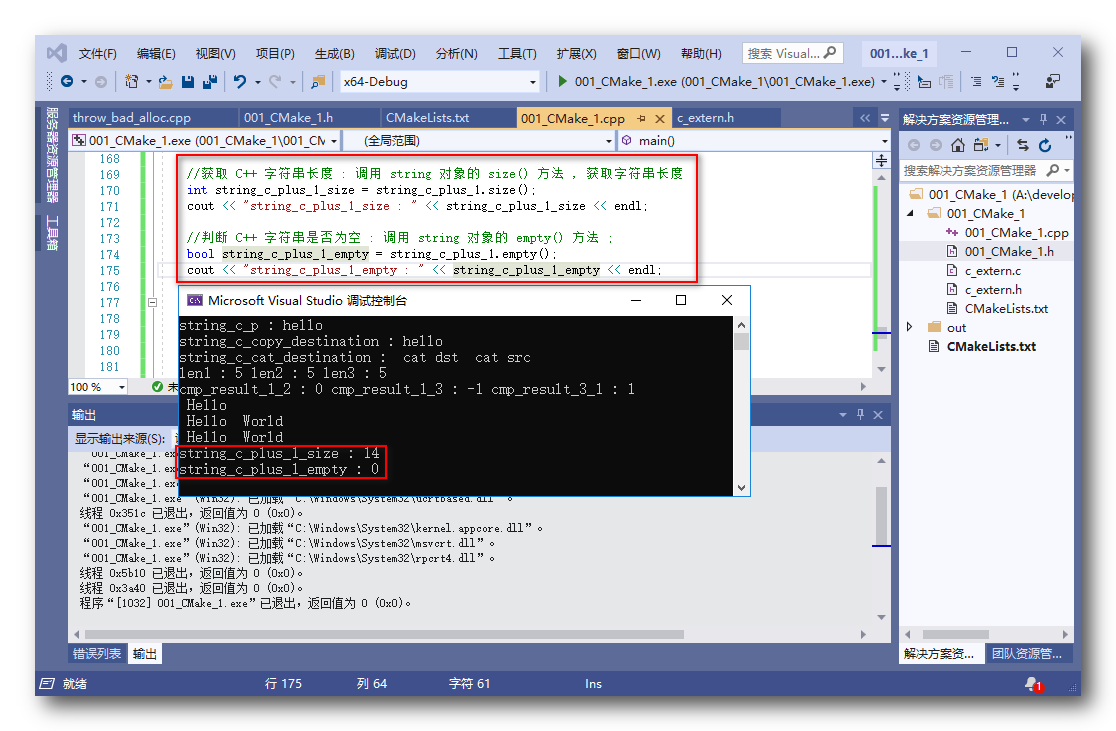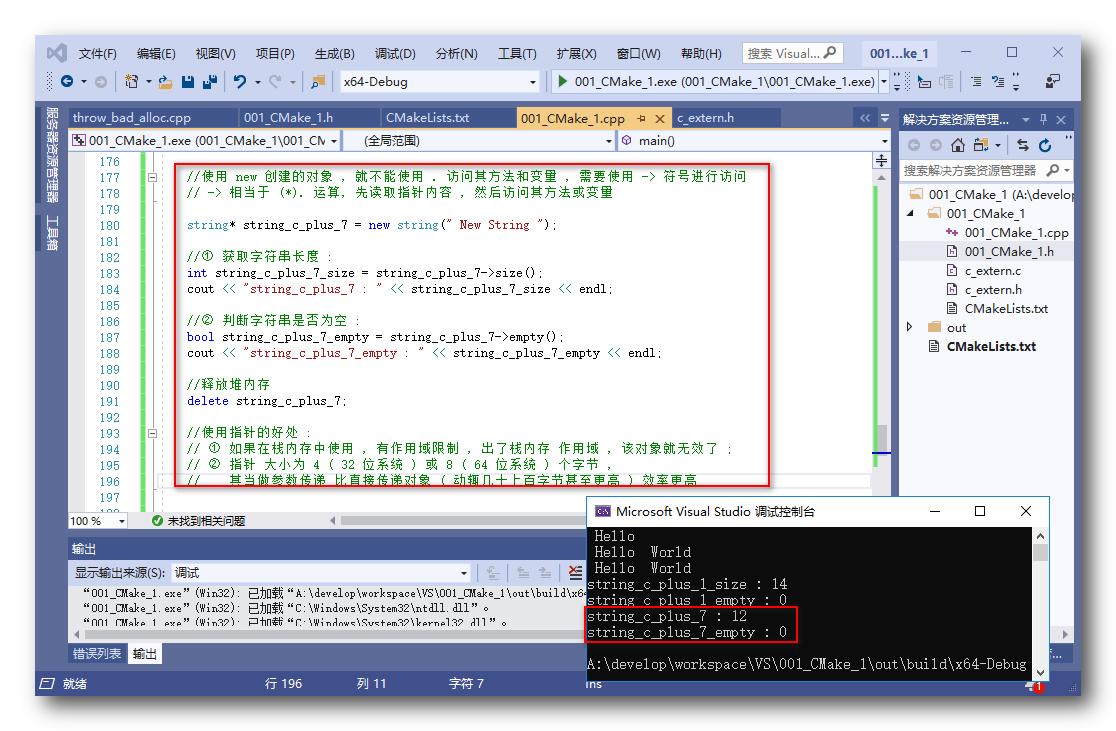【C++ 语言】C++字符串 ( string 类 | 创建方法 | 控制台输出 | 字符串操作 | 栈内存字符串对象 | string* )

22 篇文章 2 订阅

C++ 字符串 对象

C++ 字符串 :

• 1.string 类 : C++ 中的字符串是 string 类 对象 ;
• 2.string 类定义位置 : 该 string 类定义在 iostream 头文件中 , 不必引入另外的头文件 ;
#include <iostream>

C++ 字符串 对象 创建方法

C++ 字符串 对象 创建方法 :

• 1.直接赋值 : 直接使用 C 字符串赋值 ;
//① 使用字符串赋值
string string_c_plus_1 = " Hello ";
• 2.构造方法 : 调用 string 构造方法 创建 string 对象 ;
• ① 传入 string 参数 : string 构造方法可以传入 string 对象 ;
• ② 传入 C 字符串参数 : string 构造方法也可以传入 一个 C 字符串 ;
//② 调用构造方法 初始化字符串
string string_c_plus_2(string_c_plus_1);
string string_c_plus_3(" World ");
• 3.使用 new 分配内存 : 使用 new 为 String 对象分配一块堆内存空间 , 返回 string * 类型的指针 ;
//③ 使用 new 申请的内存 , 需要使用 delete 释放
string *string_c_plus_4 = new string(" New ");
delete string_c_plus_4;

① 使用 new 申请的内存 , 需要使用 delete 释放 ;
② 使用 new [] 申请的数组 , 需要使用 delete[] 释放 ;
③ 使用 malloc 申请的内存 , 需要使用 free 释放 ;

C++ 字符串 对象 输出到控制台

C++ 字符串 对象 输出到控制台 :

• 1.不能直接使用 cout 输出 : 下面的用法是错误的 ; 使用 cout 不能直接将 string 对象输出 ;
//这种用法是错误的
string string_c_plus_1 = " Hello ";
cout << string_c_plus_1 << endl;
• 2.输出方法 : 需要将 string 对象转成 C 字符串 , 然后再输出 :
//调用 string 对象的 c_str() 方法 , 获取其 C 字符串 , 然后再输出到控制台
string string_c_plus_1 = " Hello ";
cout << string_c_plus_2.c_str() << endl;

C++ 字符串 拼接

C++ 字符串拼接 :

• 1.使用 “+” 符号拼接 : 这里的加号进行了操作符重载 , 字符串之间相加代表了字符串的拼接操作 ;
//① "+" : 操作符重载 , 重新定义了 加号运算符的行为 ,
//		这里加号可以实现字符串拼接 , 与 Java 类似
string string_c_plus_5 = string_c_plus_1 + string_c_plus_3;
• 2.调用 string 对象的 append 方法 : 调用 string 对象的 append() 方法 , 传入要拼接的字符串 ;
//② 调用 string 对象的 append 方法 , 拼接字符串
string string_c_plus_6 = string_c_plus_1.append( string_c_plus_3 );
• 3.代码示例 :
//C++ 字符串拼接
//① "+" : 操作符重载 , 重新定义了 加号运算符的行为 , 这里加号可以实现字符串拼接 , 与 Java 类似
//② 调用 string 对象的 append 方法 , 拼接字符串
string string_c_plus_5 = string_c_plus_1 + string_c_plus_3;
string string_c_plus_6 = string_c_plus_1.append( string_c_plus_3 );
• 4.运行结果 :
Hello  World
Hello  WorldC++ 字符串 方法调用 ( 栈内存对象 )

C++ 字符串 方法调用 : 该字符串 ( string ) 对象 在栈内存中 ;

• 1.获取字符串长度 : 调用 string 对象的 size() 方法 , 即可返回字符串长度 ;
//① 使用字符串赋值
string string_c_plus_1 = " Hello ";
//获取 C++ 字符串长度 : 调用 string 对象的 size() 方法 , 获取字符串长度
int string_c_plus_1_size = string_c_plus_1.size();
• 2.判断字符串是否为空 : 调用 string 对象的 empty() 方法 , 判断字符串是否为空 ;
//① 使用字符串赋值
string string_c_plus_1 = " Hello ";
//判断 C++ 字符串是否为空 : 调用 string 对象的 empty() 方法 ;
bool string_c_plus_1_empty = string_c_plus_1.empty();
• 3.代码示例 :
//使用字符串赋值
string string_c_plus_1 = " Hello ";

//获取 C++ 字符串长度 : 调用 string 对象的 size() 方法 , 获取字符串长度
int string_c_plus_1_size = string_c_plus_1.size();
cout << "string_c_plus_1_size : " << string_c_plus_1_size << endl;

//判断 C++ 字符串是否为空 : 调用 string 对象的 empty() 方法 ;
bool string_c_plus_1_empty = string_c_plus_1.empty();
cout << "string_c_plus_1_empty : " << string_c_plus_1_empty << endl;
• 4.运行结果 :
string_c_plus_1_size : 14
string_c_plus_1_empty : 0C++ 字符串 方法调用 ( 堆内存对象 )

C++ 字符串 堆内存对象 方法调用 :

• 1.堆内存对象创建 : 使用 new 创建的 string 对象 需要在堆内存中为其分配内存 , 返回的是 string* 指针类型 ;
//使用 new 创建的对象 , 就不能使用 . 访问其方法和变量 , 需要使用 -> 符号进行访问
// -> 相当于 (*). 运算, 先读取指针内容 , 然后访问其方法或变量

string* string_c_plus_7 = new string(" New String ");
• 1.获取字符串长度 : 调用 string 对象的 size() 方法 , 即可返回字符串长度 ; 需要使用 -> 代替 . 操作 ;
//① 获取字符串长度 :
int string_c_plus_7_size = string_c_plus_7->size();
• 2.判断字符串是否为空 : 调用 string 对象的 empty() 方法 , 判断字符串是否为空 ; 需要使用 -> 代替 . 操作 ;
//② 判断字符串是否为空 :
bool string_c_plus_7_empty = string_c_plus_7->empty();
• 3.使用指针的好处 :
• ① 扩大作用域 : 如果在栈内存中使用 , 有作用域限制 , 出了栈内存 作用域 , 该对象就无效了 ;
• ② 方便参数传递 : 指针 大小为 4 ( 32 位系统 ) 或 8 ( 64 位系统 ) 个字节 , 其当做参数传递 比直接传递对象 ( 动辄几十上百字节甚至更高 ) 效率更高 ;
• 4.代码示例 :
//使用 new 创建的对象 , 就不能使用 . 访问其方法和变量 , 需要使用 -> 符号进行访问
// -> 相当于 (*). 运算, 先读取指针内容 , 然后访问其方法或变量

string* string_c_plus_7 = new string(" New String ");

//① 获取字符串长度 :
int string_c_plus_7_size = string_c_plus_7->size();
cout << "string_c_plus_7 : " << string_c_plus_7_size << endl;

//② 判断字符串是否为空 :
bool string_c_plus_7_empty = string_c_plus_7->empty();
cout << "string_c_plus_7_empty : " << string_c_plus_7_empty << endl;

//释放堆内存
delete string_c_plus_7;

//使用指针的好处 :
// ① 如果在栈内存中使用 , 有作用域限制 , 出了栈内存 作用域 , 该对象就无效了 ;
// ② 指针 大小为 4 ( 32 位系统 ) 或 8 ( 64 位系统 ) 个字节 ,
//	  其当做参数传递 比直接传递对象 ( 动辄几十上百字节甚至更高 ) 效率更高
• 5.运行结果 :
string_c_plus_7 : 12
string_c_plus_7_empty : 0C / C++ 字符串 完整代码示例

// 001_CMake_1.cpp: 定义应用程序的入口点。
//

#include "001_CMake_1.h"
#include "c_extern.h"

using namespace std;

//定义方法接收 int& 引用类型变量
//并在方法中修改该变量的值
void quote(int& b) {
b = 888;
}

int main()
{
cout << "Hello CMake。" << endl;

//1. C C++ 兼容
//博客地址 : https://hanshuliang.blog.csdn.net/article/details/98840708
//调用 c_extern.h 头文件中定义的方法
//该方法定义在了 C 语言文件中

//2. 引用数据类型
//博客地址 : https://hanshuliang.blog.csdn.net/article/details/99239635
//代码 展示 流程 :
//① 定义 普通 类型 变量
int a = 8;
//② 定义 引用类型变量, 格式 : 类型名称& 变量名 = 对应类型变量名称 ;
int& b = a;
//③ 调用函数传入引用类型参数 : 将引用类型传给接收引用类型的方法
quote(b);
//④ 打印引用数据类型的修改结果 , 结果是 b 被修改成了 888
cout << b << endl;

//引用数据类型定义与使用 :
// ① 引用数据类型定义 : 类型名称& 变量名 = 对应类型变量名称 ;
// ② 引用数据类型的使用方法 : 直接当做原来的变量使用即可, 可以替换原来变量的位置使用

//引用类型解析 :
// ① int& 是引用数据类型 , b 是 a 的引用
// ② 分配一块内存存放 int 类型数据 8 , 将该内存赋予一个别名 a
// ③ 同时又给该内存赋予另外一个别名 b

//3. 字符串使用
//C 字符串 表示方法 :
// ① 字符数组 : 本质是 字符 数组 char[] , 这里注意字符数组要以 NULL 或 '\0' 结尾;
char string_c[] = { 'h', 'e', 'l', 'l', 'o', '\0' };
// ② 指针 : 使用指针形式表示字符串 , 默认末尾增加 '\0' ;
char* string_c_p = "hello";

//字符串打印 :
// ① 打印字符串 , cout 后的 << 后可以打印 字符串 , 也可以打印变量
// ② 输出 cout << 字符串或变量1 << 字符串或变量2 ... << endl 可以拼接 输出信息
cout << "string_c : " << string_c << endl;
cout << "string_c_p : " << string_c_p << endl;

//C 语言中的字符串操作
//拷贝字符串
char string_c_copy_destination;
char string_c_copy_source[] = { 'h', 'e', 'l', 'l', 'o', '\0' };
// ① 参数 : strcpy 方法是拷贝字符串的方法 , 第一个参数是目标字符串 , 第二个参数是源字符串
// ② 作用 : 该方法是将 源字符串 拷贝到 目标字符串中
strcpy(string_c_copy_destination, string_c_copy_source);
// ③ 打印拷贝结果 :
cout << "string_c_copy_destination : " << string_c_copy_destination << endl;

//拼接字符串
//① 定义目标字符串 : 拼接字符串的目标字符串的大小一定要大于等于要拼接的两个字符串大小之和, 否则会报错
char string_c_cat_destination = " cat dst ";
char string_c_cat_source[] = " cat src ";
//② 拼接字符串方法参数 : 第一个参数是目标字符串 , 第二个参数是源字符串
//③ 目标字符串大小 : 注意 目标字符串的 大小一定要大于 两个字符串实际大小
strcat(string_c_cat_destination, string_c_cat_source);
//④ 打印字符串拼接结果 :
cout << "string_c_cat_destination : " << string_c_cat_destination << endl;

//获取字符串长度
//① 参数 : 传入要获取的字符串 , 该长度不含 '\0' 结尾标志
//② 作用 : 获取实际的字符串长度 , 即自动识别 '\0' 位置 , 获取其长度 , 与所占用的内存大小无关
char string_c_len[] = { 'h', 'e', 'l', 'l', 'o', '\0' };
char string_c_len2 = { 'h', 'e', 'l', 'l', 'o', '\0' };
char * string_c_len3 = "hello";

//① 字符数组长度 , 测量从开始到 '\0' 的长度, 不包括 '\0'
int len1 = strlen(string_c_len);
//② 指定大小的字符数组长度 , 结果不是指定的大小的值 , 获取的是实际字符串长度
int len2 = strlen(string_c_len2);
//③ 指针表示的字符串 , 其获取的大小是实际字符串大小, 不包含自动添加的 '\0'
int len3 = strlen(string_c_len3);
//④ 打印 三个字符串大小
cout << "len1 : " << len1
<< " len2 : " << len2
<< " len3 : " << len3
<< endl;

//字符串比较
// ① 参数说明 : 参数是需要比较的两个字符串 , 第一个参数 str1 , 第二个参数 str2
// ② 对比规则 : str1 和 str2 两个字符串 , 从左到右 逐个对比 ASCII 码 大小 ;
//		a. 如果 str1 等于 str2 , 返回 0;
//		b. 如果 str1 > str2 , 返回值 大于 0 ;
//		c. 如果 str1 < str2 , 返回值 小于 0 ;

//定义需要比较的字符串
char* string_c_comp_1 = "Hello";
char* string_c_comp_2 = "Hello";
char* string_c_comp_3 = "hello";

// ① 两个字符串相等
int cmp_result_1_2 = strcmp(string_c_comp_1, string_c_comp_2);
// ② "Hello" 字符串 (H 对应 ASCII 72) 小于 "hello" 字符串 (h 对应 ASCII 104) , 返回值 小于 0
int cmp_result_1_3 = strcmp(string_c_comp_1, string_c_comp_3);
// ③ "hello" 字符串 (h 对应 ASCII 104) 大于 "Hello" 字符串 (H 对应 ASCII 72) , 返回值 大于 0
int cmp_result_3_1 = strcmp(string_c_comp_3, string_c_comp_1);

//输出字符串对比结果
cout << "cmp_result_1_2 : " << cmp_result_1_2
<< " cmp_result_1_3 : " << cmp_result_1_3
<< " cmp_result_3_1 : " << cmp_result_3_1
<< endl;

// C++ string 类 : 该类定义在 iostream 头文件中
//创建 string 类型对象有三种方法 :
//① 直接使用字符串赋值
//② 调用构造方法赋值
//③ 最后可以调用 new 为字符串分配一块内存

//① 使用字符串赋值
string string_c_plus_1 = " Hello ";

//② 调用构造方法 初始化字符串
string string_c_plus_2(string_c_plus_1);
string string_c_plus_3(" World ");

//上面的三种字符串不需要释放 , 因为其定义在栈内存中 , 下面使用 new 创建字符串的情况需要 delete 释放内存 ;

//③ 使用 new 申请的内存 , 需要使用 delete 释放
string *string_c_plus_4 = new string(" New ");
delete string_c_plus_4;

//使用 new [] 申请的数组 , 需要使用 delete[] 释放
//使用 malloc 申请的内存 , 需要使用 free 释放

//C++ 字符串输出
//字符串对象不能直接在 cout 中输出, cout << string string_c_plus_5 << endl; 是错误的
//cout << string_c_plus_2 << endl;
//要将 string 对象打印到控制台上, 需要将其转为 C 字符串 , char* 或 char[] 才能输出
cout << string_c_plus_1.c_str() << endl;

//C++ 字符串拼接
//① "+" : 操作符重载 , 重新定义了 加号运算符的行为 , 这里加号可以实现字符串拼接 , 与 Java 类似
//② 调用 string 对象的 append 方法 , 拼接字符串
string string_c_plus_5 = string_c_plus_1 + string_c_plus_3;
string string_c_plus_6 = string_c_plus_1.append( string_c_plus_3 );

//输出拼接的字符串
cout << string_c_plus_5.c_str() << endl;
cout << string_c_plus_6.c_str() << endl;

//获取 C++ 字符串长度 : 调用 string 对象的 size() 方法 , 获取字符串长度
int string_c_plus_1_size = string_c_plus_1.size();
cout << "string_c_plus_1_size : " << string_c_plus_1_size << endl;

//判断 C++ 字符串是否为空 : 调用 string 对象的 empty() 方法 ;
bool string_c_plus_1_empty = string_c_plus_1.empty();
cout << "string_c_plus_1_empty : " << string_c_plus_1_empty << endl;

//使用 new 创建的对象 , 就不能使用 . 访问其方法和变量 , 需要使用 -> 符号进行访问
// -> 相当于 (*). 运算, 先读取指针内容 , 然后访问其方法或变量

string* string_c_plus_7 = new string(" New String ");

//① 获取字符串长度 :
int string_c_plus_7_size = string_c_plus_7->size();
cout << "string_c_plus_7 : " << string_c_plus_7_size << endl;

//② 判断字符串是否为空 :
bool string_c_plus_7_empty = string_c_plus_7->empty();
cout << "string_c_plus_7_empty : " << string_c_plus_7_empty << endl;

//释放堆内存
delete string_c_plus_7;

//使用指针的好处 :
// ① 如果在栈内存中使用 , 有作用域限制 , 出了栈内存 作用域 , 该对象就无效了 ;
// ② 指针 大小为 4 ( 32 位系统 ) 或 8 ( 64 位系统 ) 个字节 ,
//	  其当做参数传递 比直接传递对象 ( 动辄几十上百字节甚至更高 ) 效率更高

return 0;
}

03-16
05-172495

01-05
03-01
04-25
02-051511
05-111815
07-16302
01-12772
03-082190
01-223106
11-123108
11-152030
01-25687
05-19189
11-282535
10-071546
09-202万+点击重新获取扫码支付余额充值